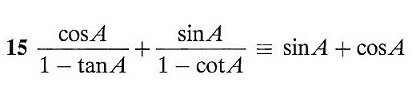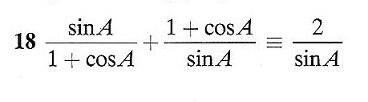# Prove these two Pythagorean identities

#### Aminta_1900

##### New member
Hi, I'm having difficulty proving these two Pythagorean identities. I'm approaching this subject for the first time and have only been practicing for the last week or so. But with the basic knowledge I have it seems that when I apply identities I am making the equations more unwieldy. So perhaps there is something simple I am not seeing. Thanks.#### Steven G

##### Elite Member
(15)...or maybe there is something you are seeing. Can we please see your work so we know what type of help you need? Since the rhs has the same denominator, namely 1, you should get the lhs to have a common denominator.

18) get all three denominators to be the same.

•stapel

#### Aminta_1900

##### New member
(15) If I look for the common denominator I get

{cos A(1 - cot A) + sin A(1 - tan A)} / {1 - cot A - tan A + tan A*cot A}

But I don't seem to see any expressions that can be simplified from here. I am awkwardly changing the cos A to sin A*cot A, or cot to 1/tan, but getting more numerators and denominators. I can only see that the tan A*cot A at the end can be changed to +1, as tan A*(1/tan A) = (tan A)/(tan A).

Till now the questions have had mostly recognizable identities, such as 1 - cos^2 A, or sec^2 - 1, but for the first time I am seeing similar expressions to these but without the second power, but don't know how to manipulate them.

(18) Likewise with the common denominator I have

(sin^2 A + 1 + 2*cos A + cos^2 A) / (sin A + sin A*cos A)

But my thoughts again would lead me to change the sin^2 A into 1 - cos^2 A, or go all obscure with (1/sec^2 A) * (cos A*tan A). Can you go from here?

But thanks for reading thus far.

#### stapel

##### Super Moderator
Staff member
(15) If I look for the common denominator I get

{cos A(1 - cot A) + sin A(1 - tan A)} / {1 - cot A - tan A + tan A*cot A}
Isn't the cotangent the reciprocal of the tangent? So shouldn't their product be simplify-able?

(18) Likewise with the common denominator I have

(sin^2 A + 1 + 2*cos A + cos^2 A) / (sin A + sin A*cos A)
Isn't there a simplification of [imath]\sin^2(x) + \cos^2(x)[/imath]?

#### Steven G

##### Elite Member
18) Multiply the 1st fraction by [1-cosA]/[1-cosA] and everything will come together.